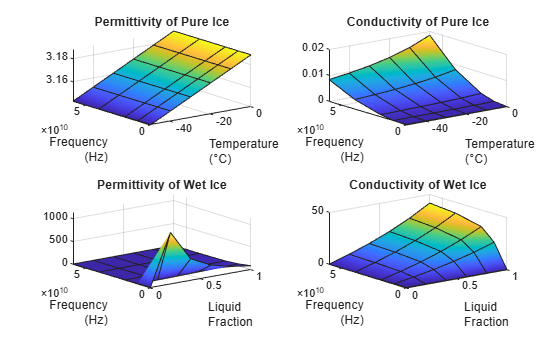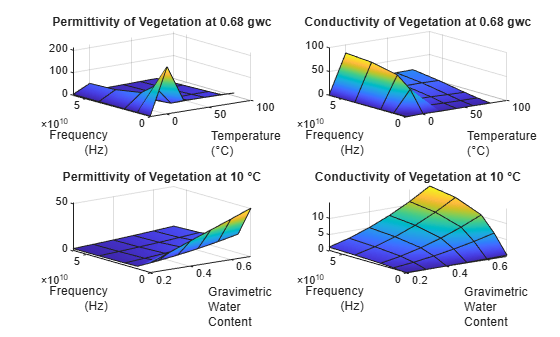# earthSurfacePermittivity

Permittivity and conductivity of earth surface materials

## Syntax

``[epsilon,sigma,complexepsilon] = earthSurfacePermittivity('pure-water',fc,temp)``
``[epsilon,sigma,complexepsilon] = earthSurfacePermittivity('dry-ice',fc,temp)``
``[epsilon,sigma,complexepsilon] = earthSurfacePermittivity('sea-water',fc,temp,salinity)``
``[epsilon,sigma,complexepsilon] = earthSurfacePermittivity('wet-ice',fc,liqfrac)``
``[epsilon,sigma,complexepsilon] = earthSurfacePermittivity('soil',fc,temp,sandpercent,claypercent,specificgravity,vwc)``
``[epsilon,sigma,complexepsilon] = earthSurfacePermittivity('soil',___,bulkdensity)``
``[epsilon,sigma,complexepsilon] = earthSurfacePermittivity('vegetation',fc,temp,gwc)``

## Description

The `earthSurfacePermittivity` function computes electrical characteristics (relative permittivity, conductivity, and complex relative permittivity) of earth surface materials based on the methods and equations presented in ITU-R P.527 . The `earthSurfacePermittivity` function provides various syntaxes to account for characteristics germane to the specified surface material.

example

````[epsilon,sigma,complexepsilon] = earthSurfacePermittivity('pure-water',fc,temp)` calculates the electrical characteristics for pure water at the specified frequency and temperature. For pure-water, the temperature setting must be greater than 0 ℃.```

example

````[epsilon,sigma,complexepsilon] = earthSurfacePermittivity('dry-ice',fc,temp)` calculates the electrical characteristics for dry-ice at the specified frequency and temperature. For dry-ice, the temperature must be less than or equal to 0 ℃.```

example

````[epsilon,sigma,complexepsilon] = earthSurfacePermittivity('sea-water',fc,temp,salinity)` calculates the electrical characteristics for sea water at the specified frequency, temperature, and salinity. For sea-water, the temperature must be greater than –2 ℃.```

example

````[epsilon,sigma,complexepsilon] = earthSurfacePermittivity('wet-ice',fc,liqfrac)` calculates the electrical characteristics for wet ice at the specified frequency, and liquid water volume fraction. For wet-ice, the temperature is 0 ℃.```
````[epsilon,sigma,complexepsilon] = earthSurfacePermittivity('soil',fc,temp,sandpercent,claypercent,specificgravity,vwc)` calculates the electrical characteristics for soil at the specified frequency, temperature, sand percentage, clay percentage, specific gravity, and volumetric water content.```

example

````[epsilon,sigma,complexepsilon] = earthSurfacePermittivity('soil',___,bulkdensity)` sets the soil bulk density in addition to input arguments from the previous syntax.```

example

````[epsilon,sigma,complexepsilon] = earthSurfacePermittivity('vegetation',fc,temp,gwc)` calculates the electrical characteristics for vegetation at the specified frequency, temperature, and gravimetric water content. For vegetation, the temperature must be greater than or equal to –20 ℃.```

## Examples

collapse all

Compare the relative permittivity and conductivity for salt-free (zero-salinity) sea water to pure water.

Specify a carrier frequency of 9 GHz, temperature of 30℃, and salinity of zero.

```fc = 9e9; % Carrier frequency in Hz. temp = 30; salinity = 0;```

Compute the relative permittivity and conductivity.

```[epsilon_pure_water,sigma_pure_water] = earthSurfacePermittivity('pure-water',fc,temp); [epsilon_sea_water,sigma_sea_water] = earthSurfacePermittivity('sea-water',fc,temp,salinity);```

Confirm that salt-free sea water and pure water have equal relative permittivity and conductivity.

`isequal(epsilon_pure_water,epsilon_sea_water)`
```ans = logical 1 ```
`isequal(sigma_pure_water,sigma_sea_water)`
```ans = logical 1 ```

Compare the relative permittivity and conductivity for wet ice with no liquid water to dry ice at 0℃. Confirm the results differ by a negligible amount.

Specify a carrier frequency of 12 GHz.

`fc = 12e9; % Carrier frequency in Hz.`

Calculate the relative permittivity and conductivity for wet ice with zero liquid water by volume.

```liqfrac = 0; [epsilon_wet_ice_0,sigma_wet_ice_0] = earthSurfacePermittivity('wet-ice',fc,liqfrac); % Set liquid water volume fraction to 0.```

Calculate the relative permittivity and conductivity for dry ice at 0 ℃.

```temp = 0; [epsilon_dry_ice_0,sigma_dry_ice_0] = earthSurfacePermittivity('dry-ice',fc,temp); % Set temperature to 0.```

Compare the relative permittivity and conductivity for wet ice with no liquid to dry ice at 0℃. Confirm that wet ice with no liquid and dry ice at 0℃ have essentially equal relative permittivity and conductivity.

`epsilon_wet_ice_0-epsilon_dry_ice_0`
```ans = 8.8818e-16 ```
`sigma_wet_ice_0-sigma_dry_ice_0`
```ans = -9.2179e-16 ```

Plot permittivity and conductivity versus frequency for dry ice and for wet ice. For dry ice, vary the temperature. For wet ice, vary the liquid water volume fraction. Calculate the permittivity and conductivity values by using `arrayfun` to apply the `earthSurfacePermittivity` function to the elements of the arrayed inputs.

```freq = repmat([0.1,10,20,40,60]*1e9,6,1); temp = repmat((-100:20:0)',1,5); liqfrac = repmat((0:0.2:1)',1,5); [epsilon_dry_ice, sigma_dry_ice] = arrayfun(@(x,y)earthSurfacePermittivity('dry-ice',x,y),freq,temp); [epsilon_wet_ice, sigma_wet_ice] = arrayfun(@(x,y)earthSurfacePermittivity('wet-ice',x,y),freq,liqfrac);```

Display tiled surface plots across specified ranges.

```figure tiledlayout(2,2) nexttile surf(temp,freq,epsilon_dry_ice,'FaceColor','interp') title('Permittivity of Dry Ice') xlabel('Temperature (℃)') ylabel('Frequency (Hz)') nexttile surf(temp,freq,sigma_dry_ice,'FaceColor','interp') title('Conductivity of Dry Ice') nexttile surf(liqfrac,freq,epsilon_wet_ice,'FaceColor','interp') title('Permittivity of Wet Ice') xlabel('Liquid Fraction') ylabel('Frequency (Hz)') nexttile surf(liqfrac,freq,sigma_wet_ice,'FaceColor','interp') title('Conductivity of Wet Ice')```Calculate relative permittivity and conductivity for various soil mixtures as defined by textual classifications in ITU-R P.527, Table 1.

Initialize computation variables for constant values and arrayed values.

```fc = 28e9; % Frequency in Hz temp = 23; % Temperature in °C vwc = 0.5; % Volumetric water content pSand = [51.52; 41.96; 30.63; 5.02]; % Sand percentage pClay = [13.42; 8.53; 13.48; 47.38]; % Clay percentage sg = [2.66; 2.70; 2.59; 2.56]; % Specific gravity bd = [1.6006; 1.5781; 1.5750; 1.4758]; % Bulk density (g/cm^3)```

Calculate the relative permittivity and conductivity for these textual classifications: sandy loam, loam, silty loam, and silty clay. Use `arrayfun` to apply the `earthSurfacePermittivity` function to the elements of the arrayed inputs. Tabulate the results.

```[Permittivity,Conductivity] = arrayfun(@(w,x,y,z)earthSurfacePermittivity( ... 'soil',fc,temp,w,x,y,vwc,z),pSand,pClay,sg,bd); pSilt = 100 - (pSand + pClay); % Silt percentage soilType = ["Sandy Loam";"Loam";"Silty Loam";"Silty Clay"]; varNames1 = ["Soil Textual Classification";"Sand";"Clay";"Silt";"Specific Gravity";"Bulk Density"]; varNames2 = ["Soil Textual Classification";"Permittivity";"Conductivity"];```

ITU-R P.527, Table 1 specifies the sand percentage, clay percentage, specific gravity, and bulk density for soil mixtures with these soil textual classifications.

`table(soilType,pSand,pClay,pSilt,sg,bd,'VariableNames',varNames1)`
```ans=4×6 table Soil Textual Classification Sand Clay Silt Specific Gravity Bulk Density ___________________________ _____ _____ _____ ________________ ____________ "Sandy Loam" 51.52 13.42 35.06 2.66 1.6006 "Loam" 41.96 8.53 49.51 2.7 1.5781 "Silty Loam" 30.63 13.48 55.89 2.59 1.575 "Silty Clay" 5.02 47.38 47.6 2.56 1.4758 ```

The relative permittivity and conductivity for these soil textual classifications are included in this table.

`table(soilType,Permittivity,Conductivity,'VariableNames',varNames2)`
```ans=4×3 table Soil Textual Classification Permittivity Conductivity ___________________________ ____________ ____________ "Sandy Loam" 15.281 18.2 "Loam" 14.563 16.998 "Silty Loam" 13.965 16.011 "Silty Clay" 12.861 14.647 ```

Calculate relative permittivity and conductivity versus frequency for vegetation, varying gravimetric water content and temperature.

Calculate relative permittivity and conductivity for vegetation at specified settings.

```fc = 10e9; % Frequency in Hz temp = 23; % Temperature in °C gwc = 0.68; % Gravimetric water content [epsilon_veg,sigma_veg] = ... earthSurfacePermittivity('vegetation',fc,temp,gwc)```
```epsilon_veg = 20.5757 ```
```sigma_veg = 4.9320 ```

Calculate values necessary to plot permittivity and conductivity by using `arrayfun` to apply the `earthSurfacePermittivity` function to the elements of the arrayed inputs.

For a range of temperatures, calculate values to plot permittivity and conductivity versus frequency for vegetation at a 0.68 gravimetric water content.

```fc = repmat([0.1,10,20,40,60]*1e9,6,1); gwc1 = 0.68; temp1 = repmat((-20:20:80)',1,5); [epsilon_veg_gwc,sigma_veg_gwc] = ... arrayfun(@(x,y)earthSurfacePermittivity('vegetation',x,y,gwc1),fc,temp1);```

For a range of gravimetric water contents, calculate values to plot permittivity and conductivity versus frequency for vegetation at 10°C.

```temp2 = 10; gwc2 = repmat((0.2:0.1:0.7)',1,5); [epsilon_veg_tmp, sigma_veg_tmp] = ... arrayfun(@(x,z)earthSurfacePermittivity('vegetation',x,temp2,z),fc,gwc2);```

Display tiled surface plots across specified ranges.

```figure tiledlayout(2,2) nexttile surf(temp1,fc,epsilon_veg_gwc,'FaceColor','interp') title('Permittivity of Vegetation at 0.68 gwc') xlabel('Temperature (℃)') ylabel('Frequency (Hz)') nexttile surf(temp1,fc,sigma_veg_gwc,'FaceColor','interp') title('Conductivity of Vegetation at 0.68 gwc') nexttile surf(gwc2,fc,epsilon_veg_tmp,'FaceColor','interp') title('Permittivity of Vegetation at 10°C') xlabel('Gravimetric Water Content') ylabel('Frequency (Hz)') nexttile surf(gwc2,fc,sigma_veg_tmp,'FaceColor','interp') title('Conductivity of Vegetation at 10°C')```## Input Arguments

collapse all

Carrier frequency in Hz, specified as a scalar in the range (0, 1e12].

Data Types: `double`

Temperature in °C, specified as a numeric scalar. Valid surfaces and associated temperature limits are indicated in this table.

SurfaceValid Temperature (℃)

pure-water

greater than 0

dry-ice

less than or equal to 0

sea-water

greater than or equal to –2

soil

any numeric

vegetation

≥ –20

### Note

When the surface is wet-ice, the temperature is 0 ℃.

Data Types: `double`

Salinity of the sea water in g/Kg, specified as a nonnegative scalar.

Data Types: `double`

Liquid water volume fraction of the wet ice, specified as a numeric scalar in the range [0, 1].

Data Types: `double`

Sand percentage of the soil, specified as a numeric scalar in the range [0, 100]. The sum of `sandpercent` and `claypercent` must be less than or equal to 100.

Data Types: `double`

Clay percentage of the soil, specified as a numeric scalar in the range [0, 100]. The sum of `sandpercent` and `claypercent` must be less than or equal to 100.

Data Types: `double`

Specific gravity of the soil, specified as a nonnegative scalar. The specific gravity is the mass density of the soil sample divided by the mass density of the amount of water in the soil sample.

Data Types: `double`

Volumetric water content of the soil, specified as a numeric scalar in the range [0, 1]. For more information, see Soil Water Content.

Data Types: `double`

Bulk density, in g/cm3, of the soil, specified as a nonnegative scalar. For more information, see Soil Water Content.

Data Types: `double`

Gravimetric water content of the vegetation, specified as a numeric scalar in the range [0, 0.7]. For more information, see Soil Water Content.

Data Types: `double`

## Output Arguments

collapse all

Relative permittivity of the earth surface, returned as a nonnegative scalar.

Conductivity of the earth surface in Siemens per meter (S/m), returned as a nonnegative scalar.

Complex relative permittivity of the earth surface, returned as a complex scalar calculated by

`complexepsilon` = `epsilon` – 1i `sigma` / (2π`fc`ε0),

where ε0 = 8.854187817e-12 Farads per meter (F/m). ε0 is the electric constant for the permittivity of free space.

collapse all

### ITU Terrain Materials

ITU-R P.527  presents methods and equations to calculate complex relative permittivity at carrier frequencies up to 1,000 GHz for these common earth surface materials.

• Water

• Sea Water

• Dry or Wet Ice

• Dry or Wet Soil (combination of sand, clay, and silt)

• Vegetation (above and below freezing)

As described in ITU-R P.527, specific textural classification applies to these mixtures of sand, clay, and silt in soil with associated specific gravities and bulk densities.

Soil Designation Textural ClassSandy LoamLoamSilty LoamSilty Clay

% Sand

51.52

41.96

30.63

5.02

% Clay

13.42

8.53

13.48

47.38

% Silt

35.06

49.51

55.89

47.60

Specific gravity (ρs)

2.66

2.70

2.59

2.56

Bulk Density (ρb) in g/cm3

1.6006

1.5781

1.5750

1.4758

### Soil Water Content

Soil water content is expressed on a gravimetric or volumetric basis. Gravimetric water content, `gwc`, is the mass of water per mass of dry soil. Volumetric water content, `vwc`, is the volume of liquid water per volume of soil. The bulk density, `bulkdensity`, is the ratio of the dry soil weight to the volume of the soil sample. The relationship between `gwc` and `vwc` is `vwc` = `gwc``bulkdensity`. When bulk density is not specified, the value of `bulkdensity` is computed by using ITU-R P.527, Equation 36:

`bulkdensity` = 1.07256 + 0.078886 ln(pSand) + 0.038753 ln(pClay) + 0.032732 ln(pSilt),

where

 ITU-R P.527-5. "Electrical characteristics of the surface of the Earth." International Telecommunications Union - Radiocommunications Sector (ITU-R). August 2019.